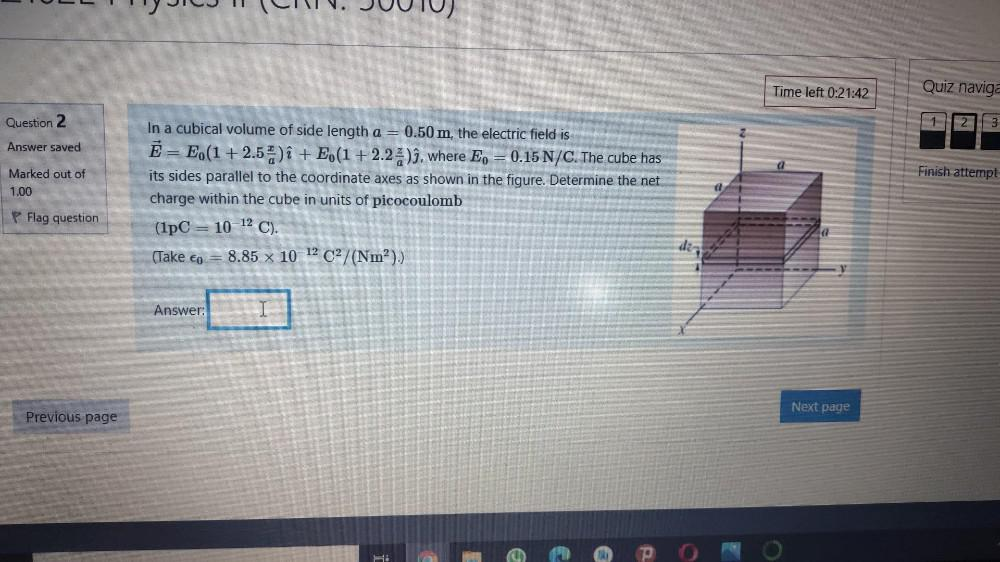Question:

# Time left 0:21:42 Quiz naviga Question 2 1 2. 3 Answer saved Finish attempt Marked out of 1.00 In a cubical volume of side lengtTime left 0:21:42 Quiz naviga Question 2 1 2. 3 Answer saved Finish attempt Marked out of 1.00 In a cubical volume of side length a = 0.50 m, the electric field is Ē = E(1 + 2.5) î + E (1+2.25)ì, where Ep = 0.15 N/C. The cube has its sides parallel to the coordinate axes as shown in the figure. Determine the net charge within the cube in units of picocoulomb (1PC = 10 12 C). (Take co = 8.85 x 10-12 C2/(Nm?).) P Flag question d Answer: I Next page Previous page